1. The big clock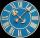The big clock hands stopped at a random moment. What is the probability that: a) a small hand showed the time between 1:00 and 3:00? b) the big hand was in the same area as a small hand in the role of a)? c) did the hours just show the time between 21:00
2. Two rectanglesI cut out two rectangles with 54 cm², 90 cm². Their sides are expressed in whole centimeters. If I put these rectangles together I get a rectangle with an area of 144 cm2. What dimensions can this large rectangle have? Write all options. Explain your calc
3. Two heights and a side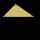Construct triangle ABC when the given side is c = 7 cm, height to side a va = 5 cm and height to side b: vb = 4 cm.
4. Glass with icecream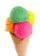We have 6 kinds of ice cream and 5 kinds of fruit. We put 3 cups of ice cream and 2 fruits into each glass. How many can unique decorated glasses be?
5. 40% volume40% volume with 104 uph (units per labor hour) 8 people working. What is the volume?
6. Regular prismThe regular four-sided prism has a base of 25 cm2 and a surface of 210 cm2. Find its volume.
7. PerformanceTwo masons with the same performance would have made of plaster for 6 days. One of them, however, has increased its daily performance by 50%. How long would take they now to make plaster together?
8. Bulbs and electricity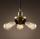In the sports hall, 875 identical light bulbs light for 2 hours. How long does it take for 100 such light bulbs to consume the same amount of electricity?
9. Angle of diagonalAngle between the body diagonal of a regular quadrilateral and its base is 60°. The edge of the base has a length of 10cm. Calculate the body volume.
10. Sand pathHow many m3 of sand is needed to fill the 1.5m wide path around a rectangular flowerbed of 8m and 14m if the sand layer is 6cm high?
11. An oilAn oil drum is cut in half. One half is used as a water trough. Use the dimensions; length 82cm, width 56cm to estimate the capacity of the water trough in liters.
12. Equilateral cylinder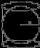A sphere is inserted into the rotating equilateral cylinder (touching the bases and the shell). Prove that the cylinder has both a volume and a surface half larger than an inscribed sphere.
13. Scientific notation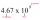Approximately 7.5x105 gallons of water flow over a waterfall each second. There are 8.6x104 seconds in 1 day. Select the approximate number of gallons of water that flow over the waterfall in 1 day.
14. ResistanceA resistor having an electrical resistance of 1.5 k ohms passes an electrical current of 0.1 A. Calculate what voltage is between the terminals of the resistor.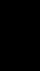The cone-shaped lampshade has a diameter of 30 cm and a height of 10 cm. How many cm2 of material will we need when we 10% is waste?
16. Triangles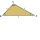Five sticks with a length of 2,3,4,5,6 cm. How many ways can you choose three sticks to form three sides of a triangle?
17. Simplify 2Simplify expression: 5ab-7+3ba-9
18. DitchDitch profile is an isosceles trapezoid with bases of length 80m and 60m. The slope of the side wall of the ditch is 80°. Calculate the ditch depth.
19. 15 teachers15 teachers teach for a combined amount of 128 days over a period of 64 days. What is this expressed as a percentage?
20. Area of iso-trapFind the area of an isosceles trapezoid if the lengths of its bases are 16 cm and 30 cm, and the diagonals are perpendicular to each other.

Do you have an interesting mathematical word problem that you can't solve it? Submit math problem, and we can try to solve it.

We will send a solution to your e-mail address. Solved examples are also published here. Please enter the e-mail correctly and check whether you don't have a full mailbox.

Please do not submit problems from current active competitions such as Mathematical Olympiad, correspondence seminars etc...